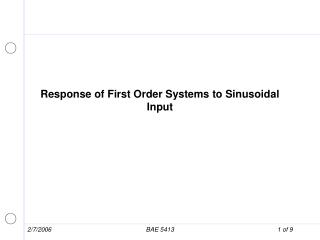DownloadDownload PresentationResponse of First Order Systems to Sinusoidal Input

# Response of First Order Systems to Sinusoidal Input

Télécharger la présentation## Response of First Order Systems to Sinusoidal Input

- - - - - - - - - - - - - - - - - - - - - - - - - - - E N D - - - - - - - - - - - - - - - - - - - - - - - - - - -
##### Presentation Transcript

1. First Order System - Sinusoidal Input Consider the following first order system: Determine the response of the system if input is a sinusoidal: Which may be transformed to: the system response, O(s), is then: Solving then for a,b, and c: BAE 5413

2. First Order System - Sinusoidal Input To solve for a, multiply by s + 1/t , and let s = -1/t To solve for b, multiply by s + iw, and let s = -iw BAE 5413

3. First Order System - Sinusoidal Input c will be the complex conjugate of b and is: Using the solution in the S&C for a sinusoidal function, the solution becomes: ,r = 0 Where: BAE 5413

4. First Order System - Sinusoidal Input The complete solution is then: It is important to note that the solution is made-up of a transient (the first term) and a non-transient part (the second term). Consider simply substuting iw for s in the original transfer function and solving for Note, this gives us the non-transient solution for a unit sine input BAE 5413

5. Plotting Frequency response BAE 5413

6. Plotting Frequency response BAE 5413

7. Plotting Frequency response BAE 5413

8. Adding terms of Frequency Response We can simply add terms on the Bode Magnitude plot and on the Bode Phase plot to get total response BAE 5413